• 基于BP神经网络的手写数字识别系统，程序使用python语言编写，训练数据集为mnist；交互界面使用PYQT编写，用户可在画板通过鼠标书写数字作为识别对象。
• 4使用minist数据集，也可以导入其他手写数字数据集
• 基于MATLAB（2016）的手写数字识别系统，对手写数字进行特征提取等预处理，使用BP神经网络对特征进行训练，得到模型后分别对手写数字图片和视频进行识别，附有详细的手打注释
•机器学习
• 基于Matlab的手写数字识别系统，准确率还行，配有GUI界面
• 本文使用Tensorflow框架进行Python编程实现基于卷积神经网络的手写数字识别算法，并将其封装在一个GUI界面中，最终，设计并实现了一个手写数字识别系统。
手写数字识别算法的设计与实现
本文使用python基于TensorFlow设计手写数字识别算法，并编程实现GUI界面，构建手写数字识别系统。这是本人的本科毕业论文课题，当然，这个也是机器学习的基本问题。本博文不会以论文的形式展现，而是以编程实战完成机器学习项目的角度去描述。

项目要求：本文主要解决的问题是手写数字识别，最终要完成一个识别系统。
设计识别率高的算法，实现快速识别的系统。
1 LeNet-5模型的介绍
本文实现手写数字识别，使用的是卷积神经网络，建模思想来自LeNet-5，如下图所示：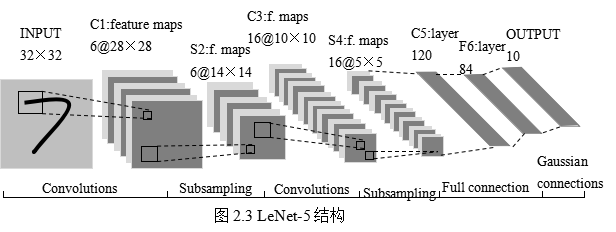这是原始的应用于手写数字识别的网络，我认为这也是最简单的深度网络。
LeNet-5不包括输入，一共7层，较低层由卷积层和最大池化层交替构成，更高层则是全连接和高斯连接。
LeNet-5的输入与BP神经网路的不一样。这里假设图像是黑白的，那么LeNet-5的输入是一个32*32的二维矩阵。同时，输入与下一层并不是全连接的，而是进行稀疏连接。本层每个神经元的输入来自于前一层神经元的局部区域(5×5)，卷积核对原始图像卷积的结果加上相应的阈值，得出的结果再经过激活函数处理，输出即形成卷积层（C层）。卷积层中的每个特征映射都各自共享权重和阈值，这样能大大减少训练开销。降采样层（S层）为减少数据量同时保存有用信息，进行亚抽样。
第一个卷积层（C1层）由6个特征映射构成，每个特征映射是一个28×28的神经元阵列，其中每个神经元负责从5×5的区域通过卷积滤波器提取局部特征。一般情况下，滤波器数量越多，就会得出越多的特征映射，反映越多的原始图像的特征。本层训练参数共6×(5×5+1)=156个，每个像素点都是由上层5×5=25个像素点和1个阈值连接计算所得，共28×28×156=122304个连接。
S2层是对应上述6个特征映射的降采样层（pooling层）。pooling层的实现方法有两种，分别是max-pooling和mean-pooling，LeNet-5采用的是mean-pooling，即取n×n区域内像素的均值。C1通过2×2的窗口区域像素求均值再加上本层的阈值，然后经过激活函数的处理，得到S2层。pooling的实现，在保存图片信息的基础上，减少了权重参数，降低了计算成本，还能控制过拟合。本层学习参数共有1*6+6=12个，S2中的每个像素都与C1层中的2×2个像素和1个阈值相连，共6×(2×2+1)×14×14=5880个连接。
S2层和C3层的连接比较复杂。C3卷积层是由16个大小为10×10的特征映射组成的，当中的每个特征映射与S2层的若干个特征映射的局部感受野（大小为5×5）相连。其中，前6个特征映射与S2层连续3个特征映射相连，后面接着的6个映射与S2层的连续的4个特征映射相连，然后的3个特征映射与S2层不连续的4个特征映射相连，最后一个映射与S2层的所有特征映射相连。此处卷积核大小为5×5，所以学习参数共有6×(3×5×5+1)+9×(4×5×5+1)+1×(6×5×5+1)=1516个参数。而图像大小为28×28，因此共有151600个连接。
S4层是对C3层进行的降采样，与S2同理，学习参数有16×1+16=32个，同时共有16×(2×2+1)×5×5=2000个连接。
C5层是由120个大小为1×1的特征映射组成的卷积层，而且S4层与C5层是全连接的，因此学习参数总个数为120×(16×25+1)=48120个。
F6是与C5全连接的84个神经元，所以共有84×(120+1)=10164个学习参数。
卷积神经网络通过通过稀疏连接和共享权重和阈值，大大减少了计算的开销，同时，pooling的实现，一定程度上减少了过拟合问题的出现，非常适合用于图像的处理和识别。
2 手写数字识别算法模型的构建
2.1 各层设计
有了第一节的基础知识，在这基础上，进行完善和改进。

输入层设计

输入为28×28的矩阵，而不是向量。

激活函数的选取

Sigmoid函数具有光滑性、鲁棒性和其导数可用自身表示的优点，但其运算涉及指数运算，反向传播求误差梯度时，求导又涉及乘除运算，计算量相对较大。同时，针对本文构建的含有两层卷积层和降采样层，由于sgmoid函数自身的特性，在反向传播时，很容易出现梯度消失的情况，从而难以完成网络的训练。因此，本文设计的网络使用ReLU函数作为激活函数。
ReLU的表达式：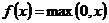卷积层设计

本文设计卷积神经网络采取的是离散卷积，卷积步长为1，即水平和垂直方向每次运算完，移动一个像素。卷积核大小为5×5。

降采样层

本文降采样层的pooling方式是max-pooling，大小为2×2。

输出层设计

输出层设置为10个神经网络节点。数字0~9的目标向量如下表所示：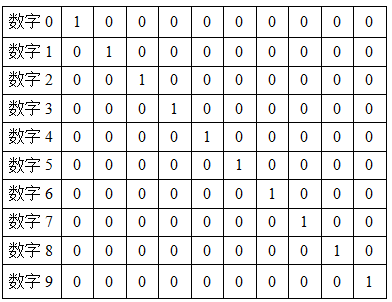2.2 网络模型的总体结构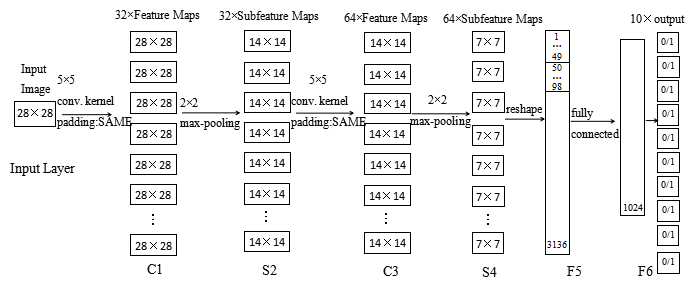其实，本文网络的构建，参考自TensorFlow的手写数字识别的官方教程的，读者有兴趣也可以详细阅读。
2.3 编程实现算法
本文使用Python，调用TensorFlow的api完成手写数字识别的算法。 注：本文程序运行环境是：Win10,python3.5.2。当然，也可以在Linux下运行，由于TensorFlow对py2和py3兼容得比较好，在Linux下可以在python2.7中运行。
#!/usr/bin/env python2
# -*- coding: utf-8 -*-
"""
Created on Fri Feb 17 19:50:49 2017

@author: Yonghao Huang
"""

#import modules
import numpy as np
import matplotlib.pyplot as plt
import tensorflow as tf
import time
from datetime import timedelta
import math
from tensorflow.examples.tutorials.mnist import input_data

def new_weights(shape):
return tf.Variable(tf.truncated_normal(shape,stddev=0.05))
def new_biases(length):
return tf.Variable(tf.constant(0.1,shape=length))
def conv2d(x,W):
def max_pool_2x2(inputx):

#import data
data = input_data.read_data_sets("./data", one_hot=True)  # one_hot means [0 0 1 0 0 0 0 0 0 0] stands for 2

print("Size of:")
print("--Training-set:\t\t{}".format(len(data.train.labels)))
print("--Testing-set:\t\t{}".format(len(data.test.labels)))
print("--Validation-set:\t\t{}".format(len(data.validation.labels)))
data.test.cls = np.argmax(data.test.labels,axis=1)   # show the real test labels:  [7 2 1 ..., 4 5 6], 10000values

x = tf.placeholder("float",shape=[None,784],name='x')
x_image = tf.reshape(x,[-1,28,28,1])

y_true = tf.placeholder("float",shape=[None,10],name='y_true')
y_true_cls = tf.argmax(y_true,dimension=1)
# Conv 1
layer_conv1 = {"weights":new_weights([5,5,1,32]),
"biases":new_biases()}
h_conv1 = tf.nn.relu(conv2d(x_image,layer_conv1["weights"])+layer_conv1["biases"])
h_pool1 = max_pool_2x2(h_conv1)
# Conv 2
layer_conv2 = {"weights":new_weights([5,5,32,64]),
"biases":new_biases()}
h_conv2 = tf.nn.relu(conv2d(h_pool1,layer_conv2["weights"])+layer_conv2["biases"])
h_pool2 = max_pool_2x2(h_conv2)
# Full-connected layer 1
fc1_layer = {"weights":new_weights([7*7*64,1024]),
"biases":new_biases()}
h_pool2_flat = tf.reshape(h_pool2,[-1,7*7*64])
h_fc1 = tf.nn.relu(tf.matmul(h_pool2_flat,fc1_layer["weights"])+fc1_layer["biases"])
# Droupout Layer
keep_prob = tf.placeholder("float")
h_fc1_drop = tf.nn.dropout(h_fc1,keep_prob)
# Full-connected layer 2
fc2_layer = {"weights":new_weights([1024,10]),
"biases":new_weights()}
# Predicted class
y_pred = tf.nn.softmax(tf.matmul(h_fc1_drop,fc2_layer["weights"])+fc2_layer["biases"])  # The output is like [0 0 1 0 0 0 0 0 0 0]
y_pred_cls = tf.argmax(y_pred,dimension=1)  # Show the real predict number like '2'
# cost function to be optimized
cross_entropy = -tf.reduce_mean(y_true*tf.log(y_pred))
# Performance Measures
correct_prediction = tf.equal(y_pred_cls,y_true_cls)
accuracy = tf.reduce_mean(tf.cast(correct_prediction,"float"))
with tf.Session() as sess:
init = tf.global_variables_initializer()
sess.run(init)
train_batch_size = 50
def optimize(num_iterations):
total_iterations=0
start_time = time.time()
for i in range(total_iterations,total_iterations+num_iterations):
x_batch,y_true_batch = data.train.next_batch(train_batch_size)
feed_dict_train_op = {x:x_batch,y_true:y_true_batch,keep_prob:0.5}
feed_dict_train = {x:x_batch,y_true:y_true_batch,keep_prob:1.0}
sess.run(optimizer,feed_dict=feed_dict_train_op)
# Print status every 100 iterations.
if i%100==0:
# Calculate the accuracy on the training-set.
acc = sess.run(accuracy,feed_dict=feed_dict_train)
# Message for printing.
msg = "Optimization Iteration:{0:>6}, Training Accuracy: {1:>6.1%}"
# Print it.
print(msg.format(i+1,acc))
# Update the total number of iterations performed
total_iterations += num_iterations
# Ending time
end_time = time.time()
# Difference between start and end_times.
time_dif = end_time-start_time
# Print the time-usage
print("Time usage:"+str(timedelta(seconds=int(round(time_dif)))))
test_batch_size = 256
def print_test_accuracy():
# Number of images in the test-set.
num_test = len(data.test.images)
cls_pred = np.zeros(shape=num_test,dtype=np.int)
i = 0
while i < num_test:
# The ending index for the next batch is denoted j.
j = min(i+test_batch_size,num_test)
# Get the images from the test-set between index i and j
images = data.test.images[i:j, :]
# Get the associated labels
labels = data.test.labels[i:j, :]
# Create a feed-dict with these images and labels.
feed_dict={x:images,y_true:labels,keep_prob:1.0}
# Calculate the predicted class using Tensorflow.
cls_pred[i:j] = sess.run(y_pred_cls,feed_dict=feed_dict)
# Set the start-index for the next batch to the
# end-index of the current batch
i = j
cls_true = data.test.cls
correct = (cls_true==cls_pred)
correct_sum = correct.sum()
acc = float(correct_sum) / num_test
# Print the accuracy
msg = "Accuracy on Test-Set: {0:.1%} ({1}/{2})"
print(msg.format(acc,correct_sum,num_test))
# Performance after 10000 optimization iterations


运行结果显示：测试集中准确率大概为99.2%。 我还写了一些辅助函数，可以查看部分识别错误的图片，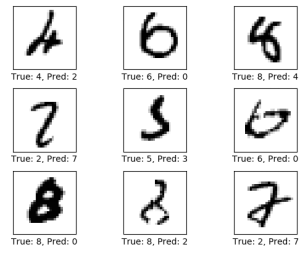还可以查看混淆矩阵，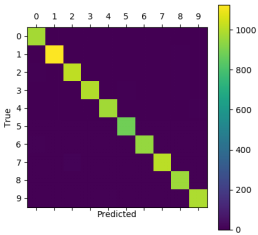2.3 实现手写识别系统
最后，将训练好的参数保存，封装进一个GUI界面中，形成一个手写识别系统。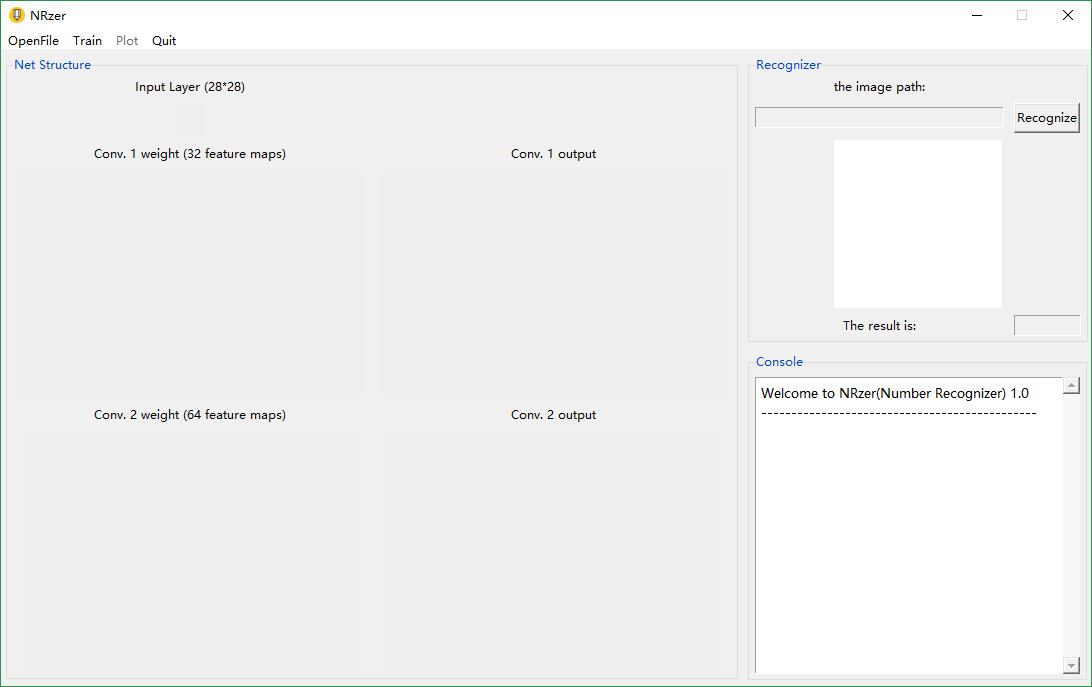系统中还添加了一点图像预处理的操作，比如灰度化，图像信息的归一化等，更贴近实际应用。 系统可进行快速识别，如下图：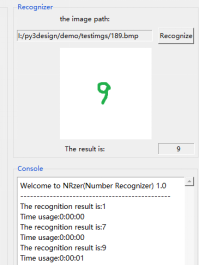3 总结
本文实现的系统其实是基于卷积神经网络的手写数字识别系统。该系统能快速实现手写数字识别，成功识别率高。缺点：只能正确识别单个数字，图像预处理还不够，没有进行图像分割，读者也可以自行添加，进行完善。
4 收获
本人之前的本科期间，虽然努力学习高数、线性代数和概率论，但是没有认真学习过机器学习，本人是2017年才开始系统学习机器学习相关知识，而且本科毕业论文也选择了相关的课题，虽然比较基础，但是认真完成后，有一种学以致用的满足感，同时也激励着我进行更深入的理论学习和实践探讨，与所有读者共勉。
================================== 2018年5月13日更新 以上是基本网络的设计与基本的实现，可满足入门学习。
相关链接:  ======================================== 2018年6月6日更新更新！！
python(TensorFlow)实现手写字符识别

此处的“手写字符”，其实指的是notMNIST数据库中的手写字符，其实和MNIST数据库是一样的。这里实现手写字符识别，主要是展示TensorFlow框架的可拓展性很强，具体来说，就是可以通过改动少部分的代码，从而实现一个新的识别功能。
NotMnist数据库
这个数据库和MNIST数据库基本一样，只是把10个数字换成了10个字母，即：A,B,C,D,E,F,G,H,I,J,K 当然，这个数据库的识别难度大一些，因为数据噪声更多一些，详情读者可以搜一搜了解一下。
实战
将NotMNIST数据库下载以后，放在本博文上述的网络中，基本不需要修改代码，直接训练，即可得到一个能识别字符的网络模型。 最后在测试集中的准确率，比MNIST的会低一些，大概为96%左右。 本文也将训练好的网络模型封装在和上述系统相似的GUI系统中，
[外链图片转存失败(img-k7xPyAio-1564543116627)(https://i.imgur.com/59M3NlD.png)] 识别效果还可以！ 同样，将卷积卷积层可视化。 [外链图片转存失败(img-tIWWgZB9-1564543116629)(https://i.imgur.com/4awe7NY.png)]
结语
TensorFlow框架可拓展性很强，只要设计好了网络，就能很容易的实现出来；同时，使用基本的CNN识别整体架构也是大同小异的，很多识别任务是通用的。当然，在具体的实践中需要得到接近完美的效果，还是要下很大功夫的！努力学习吧，加油！ （如果你/您有什么有趣的想法，可以在下面留言，如果我也感兴趣同时又有时间的话，我会尝试做一做，_）
展开全文机器学习 python Tensorflow 图像识别
• mnist手写数字项目中输入的手写数字，在机器学习入门项目mnist手写数字识别中，需要用到的输入图片，.png格式，免费共享。
• mnist手写数字项目中输入的手写数字，在机器学习入门项目mnist手写数字识别中，需要用到的输入图片，.png格式，免费共享。
• mnist手写数字项目中输入的手写数字，在机器学习入门项目mnist手写数字识别中，需要用到的输入图片，.png格式，免费共享。
• mnist手写数字项目中输入的手写数字，在机器学习入门项目mnist手写数字识别中，需要用到的输入图片，.png格式，免费共享。
• mnist手写数字项目中输入的手写数字，在机器学习入门项目mnist手写数字识别中，需要用到的输入图片，.png格式，免费共享。
• mnist手写数字项目中输入的手写数字，在机器学习入门项目mnist手写数字识别中，需要用到的输入图片，.png格式，免费共享。
• mnist手写数字项目中输入的手写数字，在机器学习入门项目mnist手写数字识别中，需要用到的输入图片，.png格式，免费共享。
• mnist手写数字项目中输入的手写数字，在机器学习入门项目mnist手写数字识别中，需要用到的输入图片，.png格式，免费共享。
• mnist手写数字项目中输入的手写数字，在机器学习入门项目mnist手写数字识别中，需要用到的输入图片，.png格式，免费共享。
• 利用深度学习的方法进行手写数字的识别，识别率高达99+
• 利用OpenCV中的KNN聚类方法来实现手写数字识别，可实时在创建的窗口画板上书写数字并进行识别。内含训练用的手写数字数据，如果缺失dll文件可以下载我的另一个资源“install文件”，其中包含了联合openVINO和CUDA...
• 这是一款自动识别手写数字的系统，能自动识别数字，编译通过
• 学习机器学习入门项目mnist手写数字识别项目中，读入手写数字图片 零到九的.png格式图片，网站上找不到相关资源，本人手写数字拍照并转格式为.png，打包pic文件。
• 手写数字识别程序 手写数字识别程序 手写数字识别程序 手写数字识别程序 手写数字识别程序 手写数字识别程序 手写数字识别程序 手写数字识别程序 手写数字识别程序
• 5000个mnist手写数字数字集，4500个训练集（Xtrain,ytrain），500个测试集（Xtest,ytest）。 每个样本X有400个特征，10种答案(1~10，10表示0)数据集
• 手写数字识别】基于支持向量机SVM实现手写数字识别matlab源码含GUI.md
• python_手写数字识别案例、手写数字图片处理 #1、手写数字识别案例 步骤： 收集数据 带有标签的训练数据集来源于trainingDigits文件夹里面所有的文件，接近2000个文件，每个文件中有32*32的二维数组，由01构成...
python_手写数字识别案例、手写数字图片处理

1、手写数字识别案例
步骤：
收集数据 带有标签的训练数据集来源于trainingDigits文件夹里面所有的文件，接近2000个文件，每个文件中有32*32的二维数组，由01构成，文件名称就是该文件数据对应的标签类型（即一个数字）。处理数据使其符合算法要求 最终我们要求是一个二维数组作为数据源，所以需要将每一个文件对应的特征数据处理成一行，即1*1024，这样所有样本集就变成了2000行的1024列的大二维数组根据以上得到的标签集合和大二维数据数据集，再确定一个k紧邻的k值，就可以作为输入条件进行训练了。给定一个测试文件，将其跟第2步一样处理成为1*1024数组，作为未知样本，然后根据knn分类算法，进行分类操作，算法同电影分类案例。 函数头：def getcls(cur,cls,dataSet,k): def getcls(cur,cls,dataSet,k) Cur：未知样本，cls：训练数据集标签集合，dataSet：训练数据集 k：最近的几个邻居
‘’’ #coding=utf-8 #手写数字识别案例 import numpy as np import os ‘’‘fnames为所有的文件名，fname为每一个文件名，vec为一个1*1024的二维数组，f表示整个文件内容，line为某一行’’’ ‘’‘函数用来根据样本集合和未知点判断最终未知点所属类别,knn’’’ def knncls(dataSet,cur,labels,k): #1、判断当前点到每个样本点距离 a=np.tile(cur,(dataSet.shape,1)) #重复cur使其dataSet数组的shape相同 #a与dataSet距离 diff=dataSet-a; diff=diff2 diff=np.sum(diff,axis=1) diff=diff0.5 #欧式距离 #对diff进行排序 indexes=np.argsort(diff) #返回值是距离在原来样本集合下标 #寻找前k个邻居的标签都是什么,确定了标签及出现次数{‘爱情’:11,‘动作’：80} dic={} for i in range(k): key=labels[indexes[i]] dic[key]=dic.get(key,0)+1 #对字典按照value进行排序，次数多的在前面 lst=sorted(dic.items(),key=lambda kv:kv,reverse=True) return lst
‘’‘创建一个训练集合20001024，未知数据就是11024’’’ def createDataSet(): #从traningset文件夹读取所有的文件，然后每个文件处理成一个1*1024的数组 fnames=os.listdir(‘D:/trainingDigits’) dataSet=np.zeros((len(fnames),1024))#训练集合二维数组 labels=[]#分类标签 i=0 for fname in fnames: #读取这个文件，然后将文件内容转为一维数组 vec=img2vec(‘D:/trainingDigits/’+fname) dataSet[i,:]=vec labels.append(fname) i+=1 return dataSet,labels #python可以返回两个值
‘’‘将图片变成一维数组’’’ def img2vec(fname): #定义一个11024的数组 vec=np.zeros((1,1024)) f=open(fname,‘r’) row=0 for line in f: for j in range(32): vec[row32+j]=line[j] #例如vec=line row+=1 return vec
if name==‘main’: num=0 i=0 k=input(“请输入k值：”) #提示用户输入k值 k=int(k) #创建训练集 dataSet,labels=createDataSet() #定义一个未知数据 tnames=os.listdir(‘D:/testDigits’) #读取testDigits文件 curs=np.zeros((len(tnames),1024))#测试集合二维数组 for tname in tnames: #读取这个文件，然后将文件内容转为一维数组 cur=img2vec(‘D:/testDigits/’+tname) curs[i,:]=cur #curs中是所有的文件内容 res=knncls(dataSet,cur,labels,k) #判断正确的个数用num记录，正确时加一 if tname==res: num+=1 accuracy=round(100*num/curs.shape,3) #计算手写数字识别的正确率，用百分率表示 print(“正确率为:{}%”.format(accuracy)) ‘’’

2、手写数字图片处理 处理问题：给定一张图片，对图片进行灰阶处理，然后进行二进制化，最终变成我们knn算法需要的样子。函数：将图片变成01
 将处理图片为二进制的函数定义在单独的一个py文件中，形成一个模块，方便别的模块进行导入。 主要的流程就是将图片打开之后，进行降噪处理，然后将其灰度化，最后设置一个阙值将其二值化保存到一个32*32的数组中
import numpy as np from PIL import Image import img2vec as pic import os ‘’‘函数用来根据样本集合和未知点判断最终未知点所属类别,knn’’’ def knncls(dataSet,cur,labels,k): #1、判断当前点到每个样本点距离 a=np.tile(cur,(dataSet.shape,1)) #a与dataSet距离 diff=dataSet-a; diff=diff2 diff=np.sum(diff,axis=1) diff=diff0.5 #欧式距离 #对diff进行排序 indexes=np.argsort(diff) #返回值是距离在原来样本集合下标 #寻找前k个邻居的标签都是什么,确定了标签及出现次数{‘爱情’:11,‘动作’：80} dic={} for i in range(k): key=labels[indexes[i]] dic[key]=dic.get(key,0)+1 #对字典按照value进行排序，次数多的在前面 lst=sorted(dic.items(),key=lambda kv:kv,reverse=True) return lst
‘’‘创建一个训练集合20001024，未知数据就是11024’’’ def createDataSet(): #从traningset文件夹读取所有的文件，然后每个文件处理成一个1*1024的数组 fnames=os.listdir(‘D:/trainingDigits’) #注意是/而不是 dataSet=np.zeros((len(fnames),1024))#训练集合二维数组 labels=[]#分类标签 i=0 for fname in fnames: #读取这个文件，然后将文件内容转为一维数组 vec=img2vec(‘D:/trainingDigits/’+fname) dataSet[i,:]=vec labels.append(fname) i+=1 return dataSet,labels
‘’‘将图片变成一维数组’’’ def img2vec(fname): #定义一个11024的数组 vec=np.zeros((1,1024)) f=open(fname,‘r’) row=0 for line in f: for j in range(32): vec[row32+j]=line[j] row+=1 return vec
if name==‘main’: #创建训练集 dataSet,labels=createDataSet() #定义一个未知数据 testfile=‘test.png’ #将图片处理成txt文件，里面是0、1 pic.picTo01(testfile) cur=img2vec(“test.txt”) res=knncls(dataSet,cur,labels,3) print(res)
附代码链接： http://localhost:8888/notebooks/cyl_day4(手写数字识别).ipynb
展开全文机器学习...